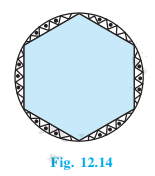# A round table cover has 6 equal designs as shown in fig 12.14. If the radius of the cover is 28 cm, find the cost of making the designs at the rate of Rs.0.35 per cm square. (Use root 3 = 1.7)Number of equal designs = 6
The radius of round table cover = 28 cm
Cost of making design = 0.35 per cm2
∠O = 360°/6 = 60°

ΔAOB is isosceles as two sides are equal. (Radius of the circle)
∴ ∠A = ∠B
Sum of all angles of triangle = 180°
∠A + ∠B + ∠O = 180°
⇒ 2 ∠A = 180° – 60°
⇒ ∠A = 120°/2
⇒ ∠A = 60°

∠A = ∠B = ∠C = 60°
Area of equilateral ΔAOB = √3/4 × (OA)2 = √3/4 × 282 = 333.2 cm2
Area of sector ACB = (60°/360°) × π r2 cm2 = 1/6 × 22/7 × 28 × 28 = 410.66 cm2

Area of design = Area of sector ACB – Area of equilateral ΔAOB

= 410.66 cm2 – 333.2 cm2 = 77.46 cm2

Area of 6 design = 6 × 77.46 cm2 = 464.76 cm2

Total cost of making design = 464.76 cm2 × 0.35 per cm2 = 162.66